# Special theory of relativity

Special Relativity (SRT) is a generalization of classical mechanics. In contrast to General Relativity, Special Relativity deals with processes observed in so called inertial frames (frames of observation without the influence of acceleration or gravity). It is based on two main observations from different experiments.

1. The speed of light is constant for all (inertial) observers, regardless of their velocities relative to each other.
2. The laws of physics are identical in all inertial reference frames.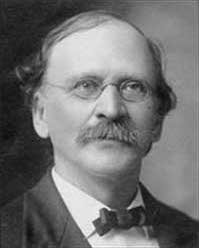Albert Michelson and Edward Morley performed the famous experiment that clinched the experimental case for Special Relativity.
It is not difficult to demonstrate that it is impossible to reconcile these conditions with a Newtonian mechanics, in which the coordinates are formed in a three-dimensional space. In SRT, each object moving in its own inertial frame has its own time, which constitutes a fourth coordinate describing its state.

The three most prominent SRT effects are time dilation, length contraction and the equivalence of mass and energy.

## Mathematics

The central equations of special relativity are the Lorentz transformations: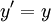These are the transformations from one coordinate systemto another system,, moving past this one at speed u, and with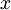and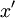axes colinear.

The Lorentz factor,occurs frequently all over special relativity and is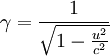, where:
•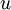is the difference in speed between the frames of reference
•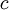is the speed of light

### Classical mechanics

When, the speed of the object in question, is low relative to,approaches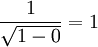, causing the Einstein-Lorentz relativity equations to be equivalent to Newton's equations. This is why classical mechanics, governed by Isaac Newton's laws of motion, works for particles at low mass and low speed. However, at higher velocities,diverges, causing relativity to be essential.

Electromagnetism, including for light and gamma radiation, where the quanta (photons) travel at light speed have no rest mass, is always relativistic.

### Universal speed limit

At the other extreme, whenapproaches,approaches. Since infinitemeans an object would have infinite kinetic energy, no object with mass can ever travel at the speed of light. Relativity does not however forbid particles from traveling faster then, rather that it is impossible to cross the speed of light barrier. Some theorists have postulated hypothetical tachyons, which would always travel faster than the speed of light. No evidence has been found for them.

## Experimental Proofs

1. Michelson-Moreley experiment
2. GPS clocks - there are two effects here and both are time dilation. The first is due to special relativity caused by the fact the satellite is in an inertial frame of reference moving past the ground. The other is due to general relativity since the craft is further out than someone on the ground of the earth's gravitational well. The overall effect is for clocks on the satellite to run faster by approximately 38 ms per day.
3. Lifetimes of fast traveling particles such as muons produced by cosmic rays (see muon)

## History

In the beginning of the last century the two assumption mentioned before where found experimentally in the form of the Maxwell equations, which describe electromagnetic waves. The general idea at that point was that any wave is carried by some medium, called the luminiferous aether. However, as the earth is moving through space, there should be a difference in the interferometrically determined light wavelength, when measured at noon, at evening and at night at the same geographic location. However the Michelson-Morley experiment did reject the aether hypothesis. With this rejection it became an inevitable fact that equations describing physics, which where fitting to a three dimensional space with a single timeframe for all observers exist. The mathematical framework and physical significance was developed by Henri Poincaré and Hendrik Lorentz. Albert Einstein gave an alternate derivation in terms of postulates. Many other scientists contributed modifications of this theory. In particular, the Irish Physicist Fitzgerald proposed that the failure of the Michelson Morley experiment was as a result of a length contraction effect. This idea was taken up by Hendrik Lorentz and shown by others to be a useful mechanism by which theory could be forced into conformance with experimental results. However, in 2005, Michael Strauss a computer engineer invalidated much of Special Relativity theory by showing clear contradictions in the theory. Special Relativity

## Spin as a relativistic effect

The relativistic extension of Quantum Mechanics, described by the Dirac Equation allows, due to the symmetry of the equation in 4-space, an additional quantum number to exist, called spin. electron spin was known from chemistry before relativity arose.